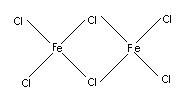# Why magnesium alkoxide is called complex as it don't consist any transition element?

Jul 28, 2017

Magnesium alkoxides are called complexes because they often exist as complex "clusters" of the alkoxide.

#### Explanation:

Alkoxide ions are good bridging ligands, that is, they can form bonds to two or more metal ions.

You see this, for example, in the dimer of ${\text{FeCl}}_{3}$where $\text{Cl}$ atoms act as bridging ligands between the two $\text{Fe}$ atoms.

Thus, four magnesium methoxide units combine in solution to form cubic structures of ["Mg"_4("OCH"_3)_8"(CH"_3"OH")_8].(Adapted from [ RSC ] Publishing - Royal Society of Chemistry)

The diagram shows only the $\text{Mg}$ atoms (blue) and the $\text{O}$ atoms (red).

One of the ${\text{OCH}}_{3}$ groups from each "Mg"("OCH"_3)_2 is at a corner of the cubic structure, and the second is outside the cube.

Two methanol molecules are also coordinated to each $\text{Mg}$ atom.